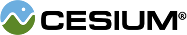#GeometryInstance

#### new Cesium.GeometryInstance(options) Core/GeometryInstance.js 59

Geometry instancing allows one `Geometry` object to be positions in several different locations and colored uniquely. For example, one `BoxGeometry` can be instanced several times, each with a different `modelMatrix` to change its position, rotation, and scale.
Name Type Description
`options` Object Object with the following properties:
Name Type Default Description
`geometry` Geometry The geometry to instance.
`modelMatrix` Matrix4 `Matrix4.IDENTITY` optional The model matrix that transforms to transform the geometry from model to world coordinates.
`id` Object optional A user-defined object to return when the instance is picked with `Scene#pick` or get/set per-instance attributes with `Primitive#getGeometryInstanceAttributes`.
`attributes` Object optional Per-instance attributes like a show or color attribute shown in the example below.
##### Example:
``````// Create geometry for a box, and two instances that refer to it.
// One instance positions the box on the bottom and colored aqua.
// The other instance positions the box on the top and color white.
var geometry = Cesium.BoxGeometry.fromDimensions({
vertexFormat : Cesium.VertexFormat.POSITION_AND_NORMAL,
dimensions : new Cesium.Cartesian3(1000000.0, 1000000.0, 500000.0)
});
var instanceBottom = new Cesium.GeometryInstance({
geometry : geometry,
modelMatrix : Cesium.Matrix4.multiplyByTranslation(Cesium.Transforms.eastNorthUpToFixedFrame(
Cesium.Cartesian3.fromDegrees(-75.59777, 40.03883)), new Cesium.Cartesian3(0.0, 0.0, 1000000.0), new Cesium.Matrix4()),
attributes : {
color : Cesium.ColorGeometryInstanceAttribute.fromColor(Cesium.Color.AQUA)
},
id : 'bottom'
});
var instanceTop = new Cesium.GeometryInstance({
geometry : geometry,
modelMatrix : Cesium.Matrix4.multiplyByTranslation(Cesium.Transforms.eastNorthUpToFixedFrame(
Cesium.Cartesian3.fromDegrees(-75.59777, 40.03883)), new Cesium.Cartesian3(0.0, 0.0, 3000000.0), new Cesium.Matrix4()),
attributes : {
color : Cesium.ColorGeometryInstanceAttribute.fromColor(Cesium.Color.AQUA)
},
id : 'top'
});``````

### Members

#### attributes : Object Core/GeometryInstance.js 116

Per-instance attributes like `ColorGeometryInstanceAttribute` or `ShowGeometryInstanceAttribute`. `Geometry` attributes varying per vertex; these attributes are constant for the entire instance.
Default Value: `undefined`

#### geometry : Geometry Core/GeometryInstance.js 75

The geometry being instanced.
Default Value: `undefined`

#### id : Object Core/GeometryInstance.js 99

User-defined object returned when the instance is picked or used to get/set per-instance attributes.
Default Value: `undefined`

#### modelMatrix : Matrix4 Core/GeometryInstance.js 87

The 4x4 transformation matrix that transforms the geometry from model to world coordinates. When this is the identity matrix, the geometry is drawn in world coordinates, i.e., Earth's WGS84 coordinates. Local reference frames can be used by providing a different transformation matrix, like that returned by `Transforms.eastNorthUpToFixedFrame`.
Default Value: `Matrix4.IDENTITY`